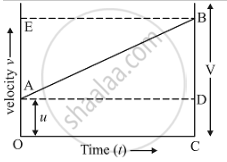Share

# Show by Using the Graphical Method That: S = U T + 1 2 a T 2 Where the Symbols Have Their Usual Meanings. - CBSE Class 9 - Science

ConceptGraphical Representation of Motion - Velocity-time Graphs

#### Question

Show by using the graphical method that: s=ut+1/2at^2 where the symbols have their usual meanings.

#### Solution

Suppose the body travels a distance (s) in time (t).In the figure, the distance travelled by the body is given by the area of the space between the velocity-time graph AB and the time axis OC, which is equal to the area of the figure OABC.
Thus: Distance travelled = Area of the trapezium OABC
But, Area of the figure OABC = Area of rectangle OADC + Area of triangle ABD
= Area of rectangle OADC + area of triangle ABD
Now, find out the area of rectangle OADC and area of triangle ABD.
= (OA) (OC)
= (u) (t)
(ii) Area of triangle ABD,
= (1/2)(t)(at)
= (1/2)at2

Distance travelled (s) is,
So, s = Area of rectangle OADC + Area of triangle ABD
s = ut + 1/2at^2
This is the second equation of motion.
Where
(s) - Displacement
(u) - Initial velocity
(a) - Acceleration
(t) - Time

Is there an error in this question or solution?

#### APPEARS IN

Solution Show by Using the Graphical Method That: S = U T + 1 2 a T 2 Where the Symbols Have Their Usual Meanings. Concept: Graphical Representation of Motion - Velocity-time Graphs.
S